Processing ......FreeComputerBooks.com Links to Free Computer, Mathematics, Technical Books all over the World

Exploring Randomness
🌠 Top Free Mathematics Books - 100% Free or Open Source!
• Title Exploring Randomness
• Author(s) Gregory J. Chaitin
• Publisher: Springer; First Edition edition (July 30, 2001)
• Hardcover/Paperback 164 pages
• eBook HTML
• Language: English
• ISBN-10: 1852334177
• ISBN-13: 978-1852334178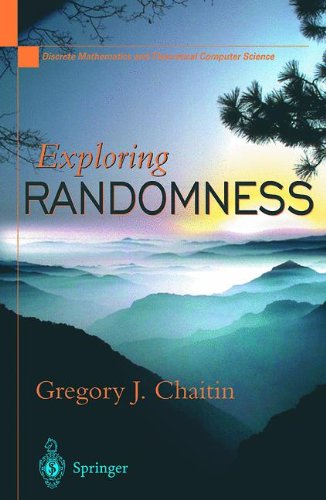Book Description

The book is devoted to a Lisp formalism for exploring the basic ideas, concepts and results on program-size complexity and random sequences. The book contains a wealth of exercises, ranging from the 'mathematical equivalent of finger warm-ups for pianists' to substantial programming projects, from open questions to questions the author cannot even formulate. Highly recommended to anyone interested in understanding algorithmic information theory through programming.

This essential companion to Chaitin's successful books The Unknowable and The Limits of Mathematics, presents the technical core of his theory of program-size complexity. The two previous volumes are more concerned with applications to meta-mathematics.

LISP is used to present the key algorithms and to enable computer users to interact with the authors proofs and discover for themselves how they work. The LISP code for this book is available at the author's Web site together with a Java applet LISP interpreter. "No one has looked deeper and farther into the abyss of randomness and its role in mathematics than Greg Chaitin. This book tells you everything hes seen. Don miss it." John Casti, Santa Fe Institute, Author of Goedel: A Life of Logic.'

• N/A
Reviews, Ratings, and Recommendations: Related Book Categories: Read and Download Links:Similar Books:
•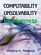Computability, Unsolvability, Randomness (Stephen G. Simpson)

The author exposits Turing's 1936 theory of computability and unsolvability, as subsequently developed by Kleene and Post. This theory is of the essence in theoretical computer science and in the study of unsolvable mathematical problems.

•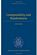Computability and Randomness (Andre Nies)

The complexity and the randomness aspect of a set of natural numbers are closely related. This book includes a detailed treatment of Turing's theory of computability and unsolvability as subsequently developed by Kleene, Post, Friedberg, etc.

•Introduction to Probability, Statistics, and Random Processes

This book introduces students to probability, statistics, and stochastic processes. It provides a clear and intuitive approach to these topics while maintaining mathematical accuracy. The book contains a large number of solved exercises.

•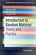Introduction to Random Matrices: Theory and Practice

This is a book for absolute beginners. The aim is to provide a truly accessible introductory account of Random Matrix theory. Most chapters are accompanied by MATLAB codes to guide readers through the numerical check of most analytical results.

•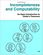Incompleteness and Computability: Gödel's Theorems

This book is an introduction to metamathematics and Gödel's Theorems. It covers recursive function theory, arithmetization of syntax, the first and second incompleteness theorem, models of arithmetic, second-order logic, and the lambda calculus.

•The Limits of Mathematics (Gregory J. Chaitin)

A course on algorithmic information theory and the epistemology of mathematics and physics. It discusses Einstein and Goedel's views on the nature of mathematics in the light of information theory, and sustains the thesis that mathematics is quasi-empirical.

•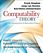Computability Theory: Introduction to Recursion Theory

Computability Theory originated with the seminal work of Gödel, Church, Turing, Kleene and Post in the 1930s. These texts provide concise, comprehensive, and authoritative introduction to contemporary computability theory, techniques, and results.

•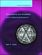Computability and Complexity: From a Programming Perspective

This book is an introduction to the basic concepts of computability, complex, and the theory of programming languages. Its goal is to build a bridge between computability and complexity theory and other areas of computer science, especially programming.

•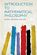Introduction to Mathematical Philosophy (Bertrand Russell)

Requiring neither prior knowledge of mathematics nor aptitude for mathematical symbolism, the book serves as essential reading for anyone interested in the intersection of mathematics and logic and in the development of analytic philosophy.

•What is Mathematics: Godel's Theorem and Around (K. Podnieks)

This accessible book gives a new, detailed and elementary explanation of the Godel incompleteness theorems and presents the Chaitin results and their relation to the da Costa-Doria results, which are given in full, but with no technicalities.

Book Categories
 :All CategoriesTop Free BooksRecent BooksMiscellaneous BooksComputer EngineeringComputer LanguagesComputer ScienceData Science/DatabasesJava and Java EE (J2EE)Linux and UnixMathematicsMicrosoft and .NETMobile ComputingNetworking and CommunicationsSoftware EngineeringSpecial TopicsWeb Programming
Other Categories